# TSA Cross-Correlation Function VI

LabVIEW 2014 Advanced Signal Processing Toolkit Help

Edition Date: June 2014

Part Number: 372656C-01

»View Product InfoDownload Help (Windows Only)

Owning Palette: Correlation and Spectral Analysis VIs

Computes the cross-correlation values of two univariate time series. Wire data to the Xt input to determine the polymorphic instance to use or manually select the instance.

Use the pull-down menu to select an instance of this VI.

 Select an instance TSA Cross-Correlation Function (Waveform)TSA Cross-Correlation Function (Array)

## TSA Cross-Correlation Function (Waveform)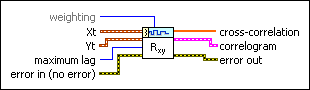weighting specifies to use the biased or unbiased weighting in the cross-correlation calculation. The default is Biased. Refer to the Details section for information about this parameter.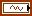Xt specifies the univariate time series.Yt specifies another univariate time series. You use this time series to perform cross-correlation with Xt.maximum lag specifies the maximum value of the lag this VI uses to compute the cross-correlation. The default is –1, which means the maximum lag equals max(M, N)–1, where M and N are the lengths of Xt and Yt, respectively.error in describes error conditions that occur before this node runs. This input provides standard error in functionality.cross-correlation returns the cross-correlation values between the two time series Xt and Yt.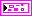correlogram returns, on an XY graph, the cross-correlation values against the lag.error out contains error information. This output provides standard error out functionality.

## TSA Cross-Correlation Function (Array)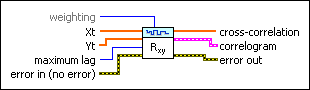weighting specifies to use the biased or unbiased weighting in the cross-correlation calculation. The default is Biased. Refer to the Details section for information about this parameter.Xt specifies the univariate time series.Yt specifies the univariate time series.maximum lag specifies the maximum value of the lag this VI uses to compute the cross-correlation. The default is –1, which means the maximum lag equals max(M, N)–1, where M and N are the lengths of Xt and Yt, respectively.error in describes error conditions that occur before this node runs. This input provides standard error in functionality.cross-correlation returns the cross-correlation values between the two time series Xt and Yt.correlogram returns, on an XY graph, the cross-correlation values against the lag.error out contains error information. This output provides standard error out functionality.

## TSA Cross-Correlation Function Details

This VI computes the cross-correlation values between two univariate time series Xt and Yt according to the following equation:where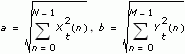Xt has length N and Yt has length M. The length of the output is N+M–1.

w is the weighting factor.

When weighting is biased, w(k) = 1.

When weighting is unbiased,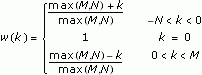## Example

Refer to the Correlation Analysis VI in the labview\examples\Time Series Analysis\TSAGettingStarted directory for an example of using the TSA Cross-Correlation Function VI.